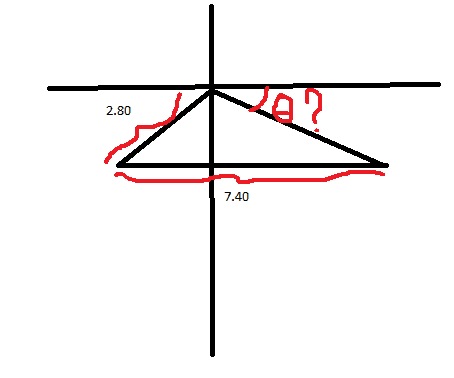# Find the angle of a triangle and x coordinateI have this triangle and I know just the two sides indicated there. How can I find angle theta?
I tried decomposing the triangle in two right triangles and using trigonometry find one side, but I can't figure how to do that using just the hypotenuse

Mentallic
Homework HelperI have this triangle and I know just the two sides indicated there. How can I find angle theta?
I tried decomposing the triangle in two right triangles and using trigonometry find one side, but I can't figure how to do that using just the hypotenuse

There isn't enough information there to solve the problem - unless the bottom length of the triangle is parallel to the x-axis as it looks to be?

If so, there's an angle within the triangle which is also equal to $\theta$ given the property that the two lines are parallel.

There isn't enough information there to solve the problem - unless the bottom length of the triangle is parallel to the x-axis as it looks to be?

If so, there's an angle within the triangle which is also equal to $\theta$ given the property that the two lines are parallel.

Yes, it is parallel. But how can I find that?

And how could I find the unknown side dimension? I would try with $\sqrt{2.80^2 + 7.40^2}$ but it isn't a right triangle

SteamKing
Staff Emeritus
Homework Helper
There is not enough information to determine the angle theta even if the base of the triangle is parallel to the upper line.

In addition to the lengths of the two sides, you would need to know the length of the third side or one of the angles of the triangle.

Redbelly98
Staff Emeritus
Homework Helper
In general: you need to know three of the six variables (3 sides plus 3 angles) commonly used to describe a triangle.

Moreover:

1. Knowing 3 angles is not enough; at least one of the knowns must be a side length.

2. Sometimes 3 knowns is not enough. Knowing 2 sides and their non-included angle can lead to 2 possible solutions, in some cases.

HallsofIvy
Homework Helper
Given that the lower side is parallel to the x-axis, that angle $\theta$ is the same as the angle in the triangle between the side labeled "7.40" and the side that is not labeled. But, as Redbelly98 said, just knowing the lengths of two sides is not sufficient to determine the triangle.

IF we were given the length of the third side, say, "x", then we could use the "cosine law" $2.8^2= 7.4^2+ x^2- 2(7.4)x cos(\theta)$ and solve that for $\theta$. But without knowing x, the best we could do is say that
$$cos(\theta)= \frac{7.4^2+ x^2- 2.8^2}{2(7.4x)}= \frac{x^2- 6.7056}{14.8x}$$
x must be such that the right side is between -1 and 1, but the angle still depends upon x.

I forgot to say that the hypotenuse was determined using the information that it moved 2.80km SW.. so SW is 45º and with that is now possible to find all the other sides. But thanks anyway for the help :)

Not enough information. You can move the bottom left or right while still maintaining the givens, but have an infinite number of triangles.